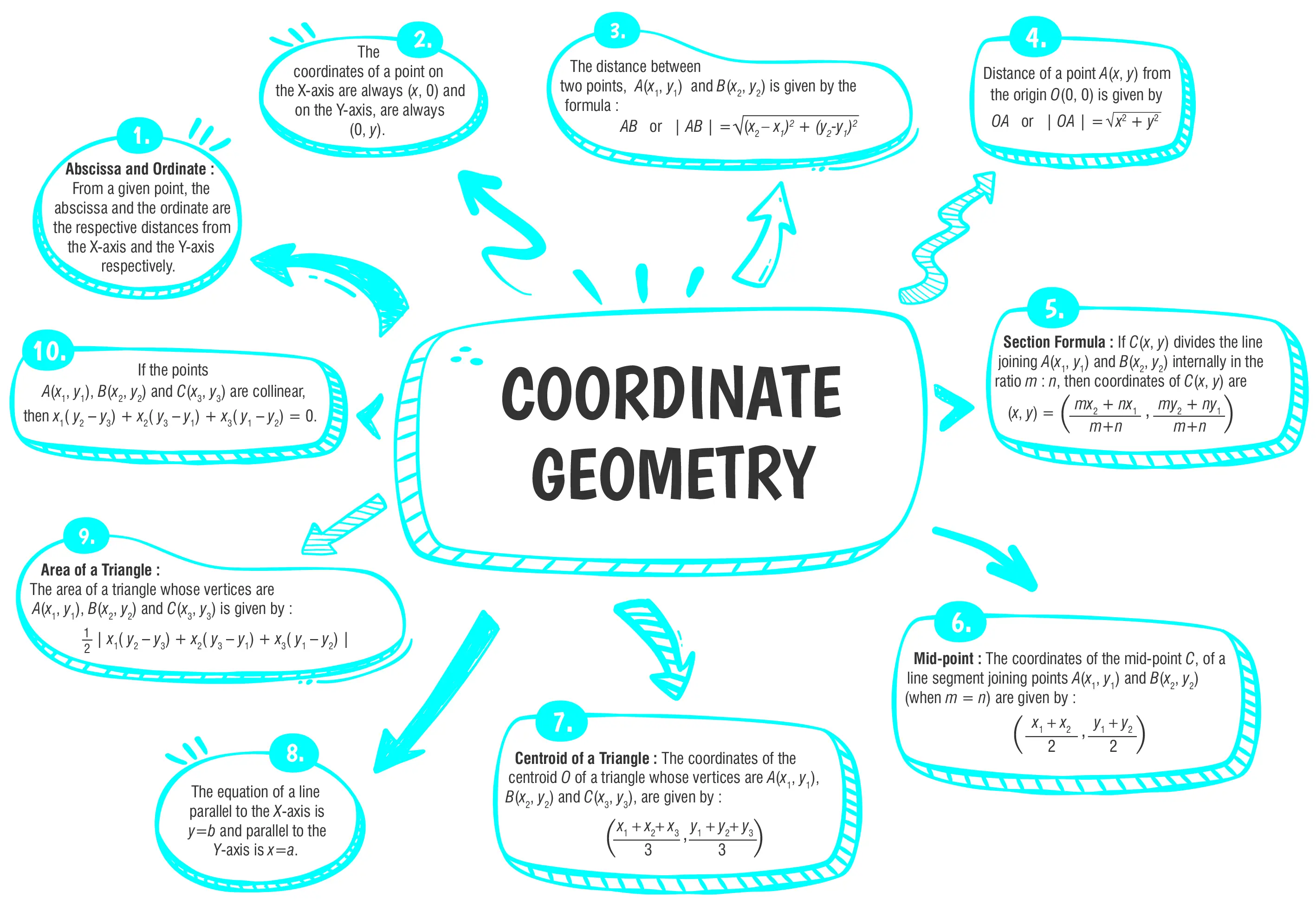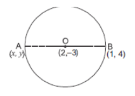Coordinate Geometry

# Class 10 Math Chapter 7 Coordinate GeometryHere are some essential Class 10 Mathematics questions for Chapter 7, Coordinate Geometry , carefully selected to assist students in preparing for the CBSE Class 10 Mathematics Examination in 2023-24. These thoughtfully curated questions cover various types of problems and aim to help students strengthen their problem-solving skills and gain a deeper understanding of the Coordinate Geometry  chapter. By practicing this diverse range of questions, students can clarify any doubts they may have and improve their performance in this chapter.

## Introduction

In this chapter, we will study concepts of coordinate geometry, graphs of linear equations, the distance formula, and the section formula for internal division.These concepts are essential in understanding the relationship between algebra and geometry.

### What are Coordinate Geometry?

Coordinate Geometry is a branch of mathematics that deals with the study of geometrical figures using a coordinate system. It involves representing points, lines, and shapes on a plane using ordered pairs of numbers (x, y) called coordinates.### Class 10 Coordinate Geometry Important Questions and Answers

#### (a) ab(b) $$\frac{1}{2}ab$$(c) $$\frac{1}{2}a^2b^2$$(d) $$\frac{1}{2} b^2$$

Ans. (b)$$\frac{1}{2}ab$$

Explanation:
Given,
A = (a, 0), O = (0, 0) and B = (0, b)
Thus, Area of △ABC
$$=\frac{1}{2} [a(0-b) + 0 (b - 0) + 0 (0 - 0)]$$
$$=-\frac{1}{2}\text{ ab sq. units}$$
$$=\frac{1}{2}\text{ ab sq. units}$$
[as area cannot be negative]

#### (a) 5 : 4(b)4 : 7(c)7 : 4(d)4 : 5

Ans. (b)4;7

Explanation:
Let, K : 1 be the ratio in which the X-axis divides the line joining (6,4) and (1,-7).Let, P be the point on the X- axis that divides the line. Then
$$P(xy)=\begin{bmatrix} \frac{k+6}{k+1},\frac{-7k+4}{k+1}\end{bmatrix}$$
$$\text{As P(x,y) lies on X-axis }P(x,0)=\begin{pmatrix} \frac{k+6}{k+1},\frac{-7k+4}{k+1} \end{pmatrix} \text{[∵ x- coordinate will be x,0]}$$
$$\Rightarrow \frac{-7k+4}{k+1}=0$$
⇒                -7K+4 = 0
⇒                        7K=4
$$\Rightarrow k= \frac{4}{7}$$
$$\text{So, the required ratio be} K : 1 =\frac{4}{7}:1= 4 : 7$$

#### Q3. Find the coordinates of point A, where AB is the diameter of a circle whose center is (2, – 3) and B is the point (1,4).

Ans. The coordinates of point A are (3,- 10)

Explanation:
Let the coordinates of point A be (x,y) and point O (2,-3) be the center, then
$$\text{By mid-point formula,} \frac{x+1}{2}=\text{2 and }\frac{y+4}{2}=-3$$
or x = 4 - 1 and y =- 6 - 4
$$\Rightarrow \text{x = 3 and y = - 10}$$
$$\therefore \text{The coordinates of point A are (3,- 10)}$$#### Q4. The x-coordinate of a point P is twice its y-coordinate. If P is equidistant from Q(2, – 5) and R(– 3, 6), find the coordinates of P.

Ans. The coordinates of point P are (16,8).

Explanation:
Let the coordinates of point P be (2y,y). since , P is equidistant from Q and R
$$\therefore PQ = PR$$
$$\Rightarrow \sqrt{(2y-2^2)+(y+5)^2}=\sqrt{(2y+3^2)(y-6^2)}$$
$$\Rightarrow (2y-2) + (y + 5)= (2y + 3) + (y - 6)$$
$$\Rightarrow 4y + 4 - 8y + + 25 + 10y = 4y+ 9 + 12y + y + 36 - 12y$$
$$\Rightarrow 2y + 29 = 45$$
$$\Rightarrow 2y = 45 - 29$$
$$\Rightarrow y=\frac{16}{2}=8$$
Hence, the coordinates of point P are (16,8).

#### Q5. Determine the ratio in which the line x – y – 2 = 0 divides the line segment joining the points A(3, – 1) and B(8, 9).

Ans. Ratio is 2 : 3

Explanation:
Given, A = (3, – 1), B = (8, 9) and x – y – 2 = 0 Let the line x – y – 2 = 0 intersect the line segment AB at R in the ratio p : 1.
Now, Rx =$$\frac{1(3)+p(8)}{p+1} and R_y =\frac{1(-1)+p(9)}{p+1}$$
$$\Rightarrow R_x =\frac{3+8p}{p+1}and R_y =\frac{-1+9p}{p+1}$$
Thus, R = $$\begin{pmatrix} \frac{3+8p}{p+1},\frac{9p-1}{p+1} \end{pmatrix}$$
Now substituting the value of R in the given equation, we get
$$\frac{3+8p}{p+1}-\frac{9p-1}{p+1}-2=0$$
$$\Rightarrow 3 + 8p – 9p + 1 – 2(p + 1) = 0$$
$$\Rightarrow 4 – p – 2p – 2 = 0$$
$$\Rightarrow 3p = 2$$
$$\Rightarrow P=\frac{2}{3}$$
Thus, the ratio is $$\frac{2}{3} : 1$$ = 2 : 3.#### CBSE Class 10 Maths Chapter wise Important Questions

Chapter No. Chapter Name
Chapter 1 Real Number
Chapter 2 Polynomials
Chapter 3 Pair of Linear Equations in Two Variables
Chapter 5 Arithmetic Progressions
Chapter 6 Triangles
Chapter 7 Coordinate Geometry
Chapter 8 Introduction to Trigonometry
Chapter 9 Some Applications of Trigonometry
Chapter 10 Circles
Chapter 11 Areas Related to Circle
Chapter 12 Surface Areas and Volumes
Chapter 13 Statistics
Chapter 14 Probability

#### Conclusion

To improve your understanding of the chapter's concepts, oswal.io offers a wide range of practice questions. Their extensive question bank is designed to help you reinforce your knowledge and enhance your problem-solving skills. By using these resources, you can strengthen your grasp of the topics and become better at solving various types of problems.

#### Q1: What is Coordinate Geometry?

Ans: Coordinate Geometry is a branch of mathematics that involves the study of geometric figures using coordinate systems. It relates algebraic equations to geometric shapes and positions them on a coordinate plane.

#### Q2: What are the coordinates of the origin?

Ans: The coordinates of the origin are (0, 0). It is the point where the x-axis and y-axis intersect.

#### Q3: How do you find the distance between two points on the coordinate plane?

Ans: The distance between two points (x1, y1) and (x, y) on the coordinate plane can be calculated using the Distance Formula: Distance = √[(x - x)² + (y - y)²]

#### Q4: State the Section Formula for internal division.

Ans: The Section Formula for internal division gives the coordinates (x, y) of a point P that divides a line segment joining two points A(x, y) and B(x, y) in a given ratio 'm:n' internally as: x = [(mx + nx)/(m + n)], y = [(my + ny)/(m + n)]

#### Q5: What is the importance of the x-axis and y-axis in the coordinate plane?

Ans: The x-axis and y-axis are essential components of the coordinate plane. The x-axis represents the horizontal axis, and the y-axis represents the vertical axis. They intersect at the origin (0, 0). The coordinates of any point on the plane are determined by its position along these two axes.

###### Copyright 2022 OSWAL PUBLISHERS Simplifying ExamsPhone:  (+91) 78959 87722
Mail: support@oswalpublishers.in